# Solving for electric potential using separation of variables

## Homework Statement

Given two two grounded concentric spherical shells with radii a,b (a<b) and a point charge q between them at a<r=R<b find:

1.The surface charge density of the point charge using the delta function, assume the charge is on the z axis

2.By using the separation of variables method find the electric potential between the shells.

## Homework Equations

I have no idea how to insert mathematical equation in the forum but the most relevant equations are the jump discontinuity in the orthogonal part of the field and the general form of the solution of the Laplace equations in spherical coordinates

## The Attempt at a Solution

In my first try I tried to solve for the whole volume at once which produced contradictions, in my second attempt I divided the volume and solved for each volume seperately but I got stuck on the orthogonality condition for the Legendre polynomials, the precise attempt is shown in the images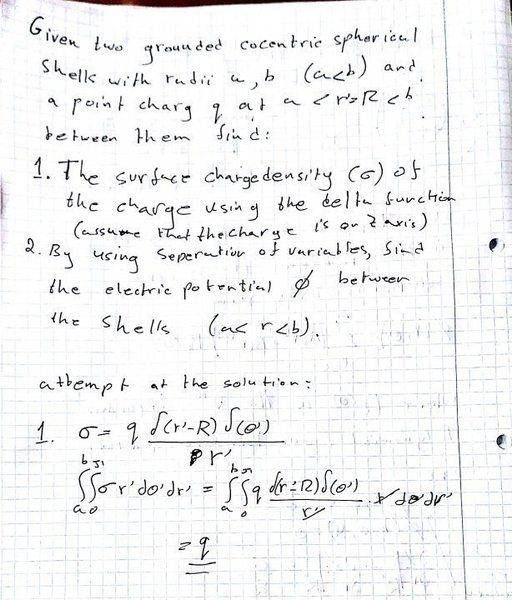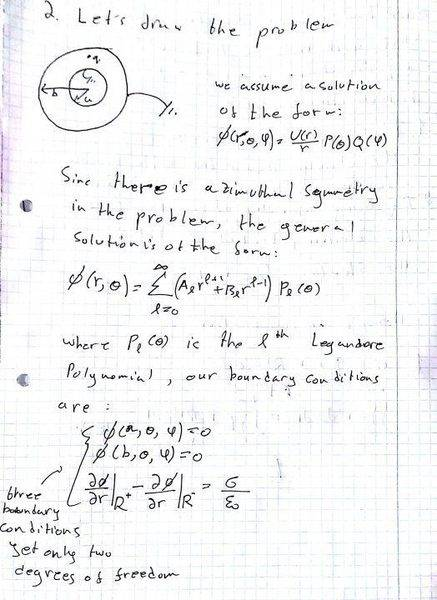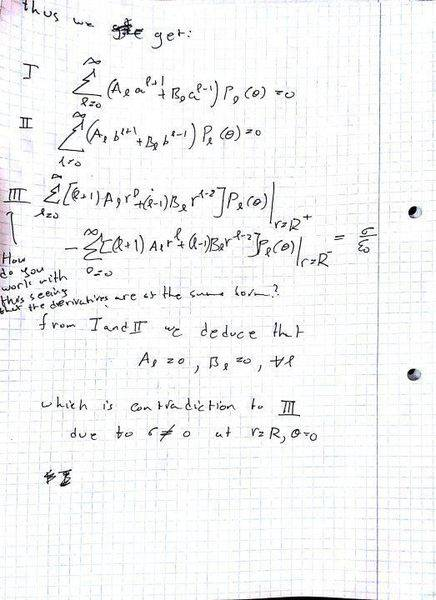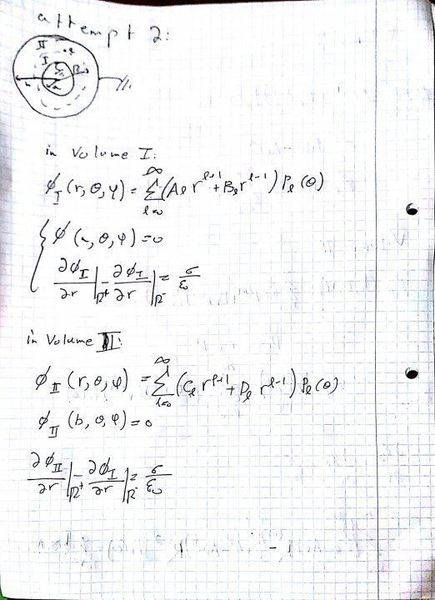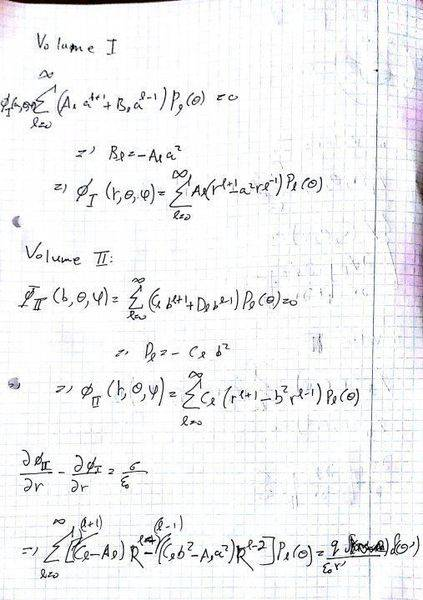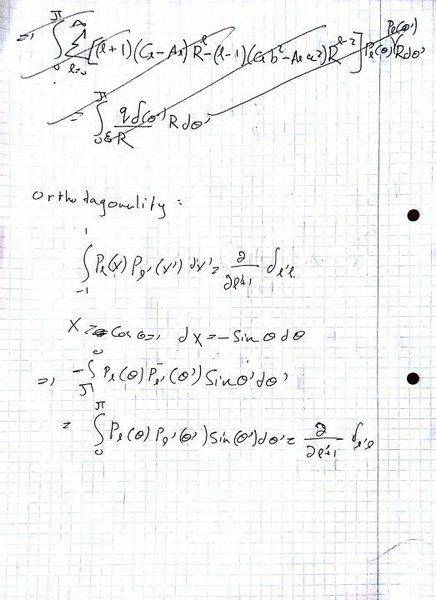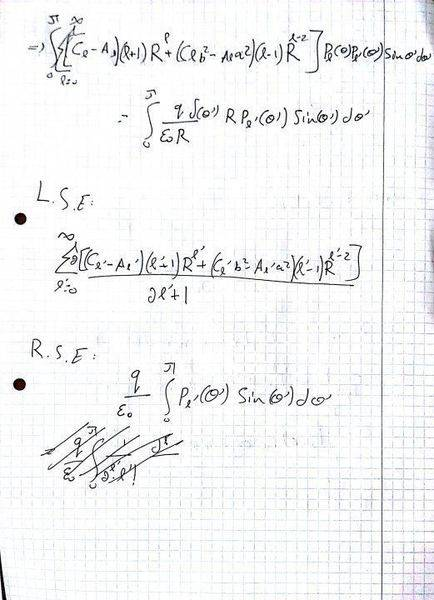TSny
Homework Helper
Gold Member
Your second approach should work. However, I noticed a few errors:

Page 1: Your expression for σ is incorrect. You want to write a surface charge density for the surface r = R. Thus, ∫σdA = q for this surface. There is no integration over r here. Think about the expression for dA for this surface.

Page 4: Your powers of r in ##\Phi_I## and ##\Phi_{II}## don't appear to be correct. For example, see eq. 14 here http://www.luc.edu/faculty/dslavsk/courses/phys301/classnotes/laplacesequation.pdf

Page 4: Check the signs in the equation at the bottom of the page for the boundary condition involving R+ and R-. Recall that ##\vec{E} = -\nabla \Phi##. ( I don't understand the equation in the middle of the page, just before "in Volume II".)

Page 7: You forgot the ##\delta_{l,l'}## that occurs when doing the integral on the left at the top of the page.

There is another boundary condition that you can use. What can you say about the continuity of ##\Phi## at r = R when ##\theta \neq 0##?